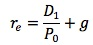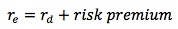### Why should I choose AnalystNotes?

Simply put: AnalystNotes offers the best value and the best product available to help you pass your exams.

##### Subject 3. Cost of Common Equity
The cost of common equity (re) is the rate of return stockholders require on common equity capital the firm obtains. It has no direct costs but is related to the opportunity cost of capital: if the firm cannot invest newly obtained equity or retained earnings and earn at least re, it should pay these funds to its stockholders and let them invest directly in other assets that do provide this return. Firms should earn on retained earnings at least the rate of return shareholders expect to earn on alternative investments with equivalent risk.

Estimating the cost of common equity is challenging due to the uncertain nature of the amount and timing of future cash flows.

The CAPM Approachwhere RF is the risk-free rate, E(RM) is the expected rate of return on the market, and βi is the stock's beta coefficient. [E(RM) - RF] is called the equity risk premium (ERP). Both E(RM) and βi need to be estimated.

For example, firm A has a βi of 0.6 for its stock. The risk-free rate, RF, is 5%. The expected rate of return on the market, E(RM), is 10%. The firm's cost of common equity is therefore calculated as 5% + (10% - 5%) x 0.6 = 8%.

There are several ways to estimate the equity risk premium.

• The historical equity risk premium approach examines the historical data of realized returns from a country's market portfolio and uses the average rate for both the market portfolio and risk-free assets. One study, cited in the textbook, found that the annualized U.S. equity risk premium relative to U.S. Treasury bills was 5.3% (geometric mean). However, there are some limitations to this approach. For example, the level of risk of the stock index and risk aversion of investors may change over time.

• The dividend discount model approach (or implied risk premium approach) analyzes how the market prices an index using the Gordon growth model:where re is the required rate of return on the market, D1 is the dividends expected next period on the index, P0 is the current market value of the equity market index, and g is the expected growth rate of the dividends.

• The survey approach is a direct one: ask a panel of financial experts for their estimates and take the mean response.

Dividend Discount Model Approachwhere D1 is the dividend expected to be paid at the end of year 1, P0 is the current price of the stock, and g is the constant growth rate of dividends.

P0 is directly known, and D1 can be predicted if the company has a stable dividend policy. However, it is difficult to establish the proper growth rate (g). One method is to forecast the firm's average future dividend payout ratio and its complement, the retention rate: g = (1.0 - Payout rate) (ROE), where ROE is the expected future rate of return on equity. Another method is to use the firm's historical growth rate, if the past growth rates are stable.

Bond Yield Plus Risk Premium Approach

Because the cost of capital of riskier cash flows is higher than that of less risky cash flows:This is a subjective, ad hoc procedure: bond yield is the interest rate on the firm's long-term debt, and the risk premium is a judgmental estimate (usually 3-5%). For example, suppose that ABC, Inc.'s interest rate on long-term debt is 10%. Assume the risk premium is 5%. ABC's cost of retained earnings is 10% + 5% = 15%.

Learning Outcome Statements

h. calculate and interpret the cost of equity capital using the capital asset pricing model approach, the dividend discount model approach, and the bond-yield-plus risk-premium approach;

CFA® 2022 Level II Curriculum, , Volume 4, Reading 33

User Comment
tomalot This is hard
fobucina Everything is a matter of practice.. Just put in the work and you'll get it
Inaganti6 This isn't rocket science but it's mind numbing to remember if you don't practice
guest this LOS is small here compared to the whole picture, I advise everyone to read it in the book.
khalifa92 the risk premium(bond yield approach) is not to be confused with the equity risk premium (CAPM):
1- equity risk premium is the difference between the cost of equity and the risk-free rate of interest.
2- risk premium is the difference between the cost of equity and the company's cost of debt.
urbanmonk Helpful clarification khalifa92, many thanks